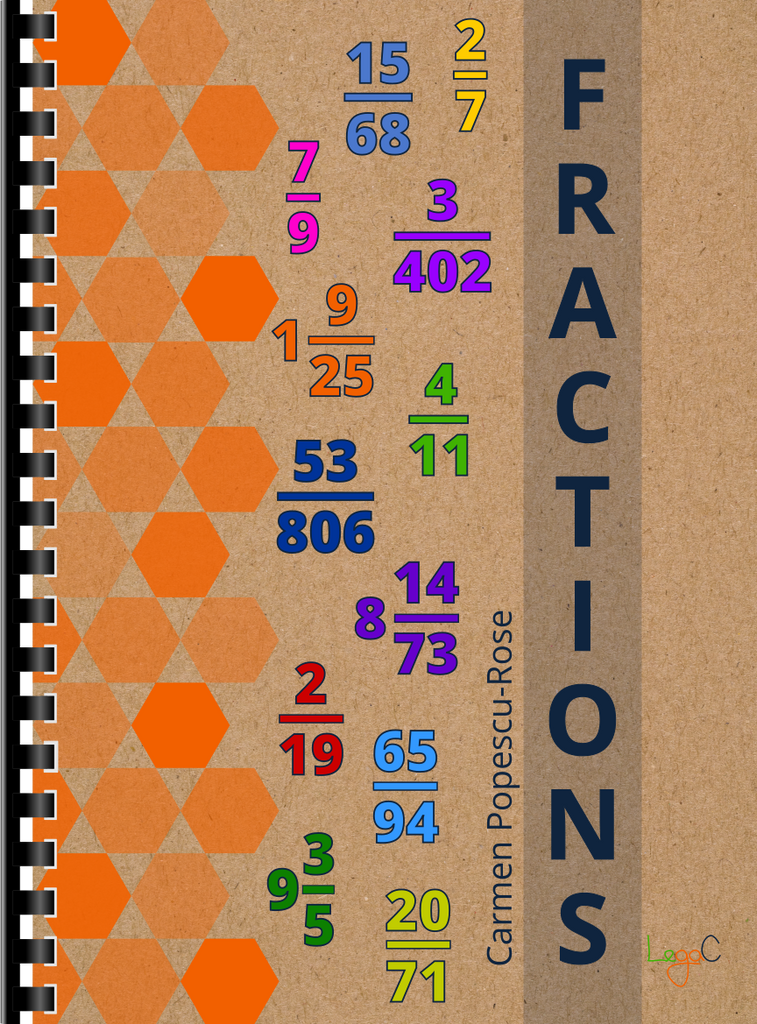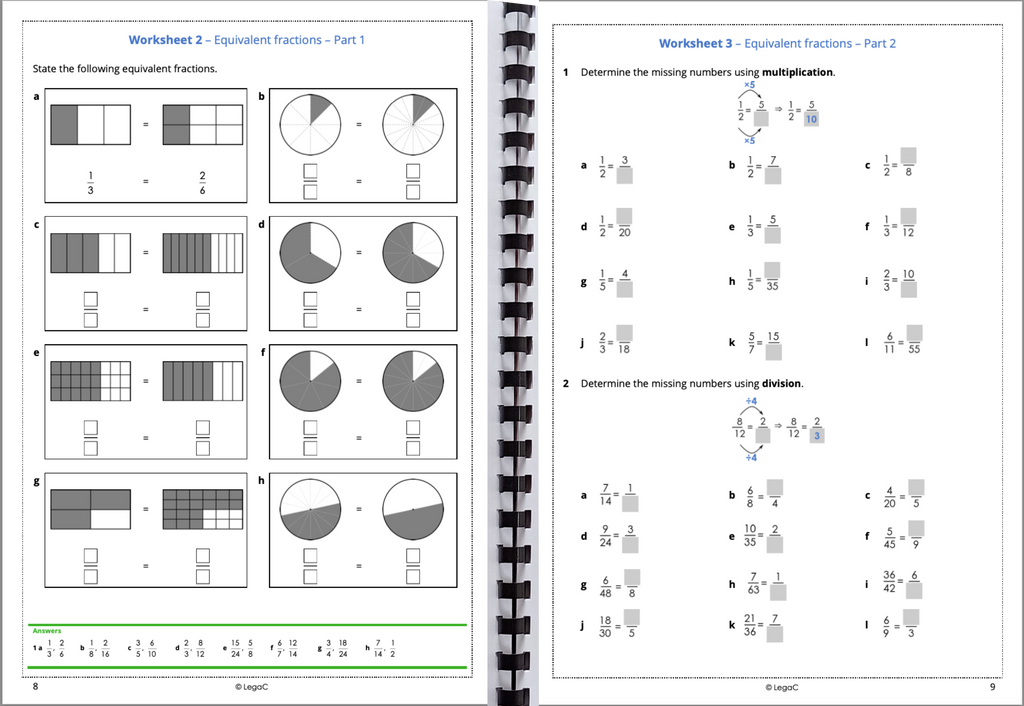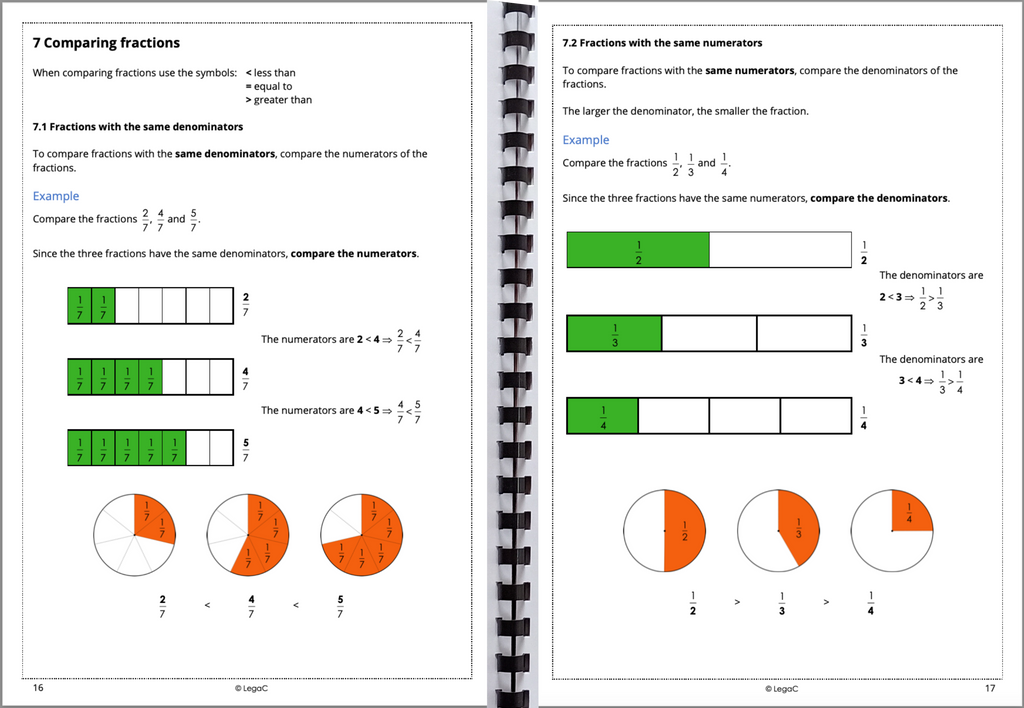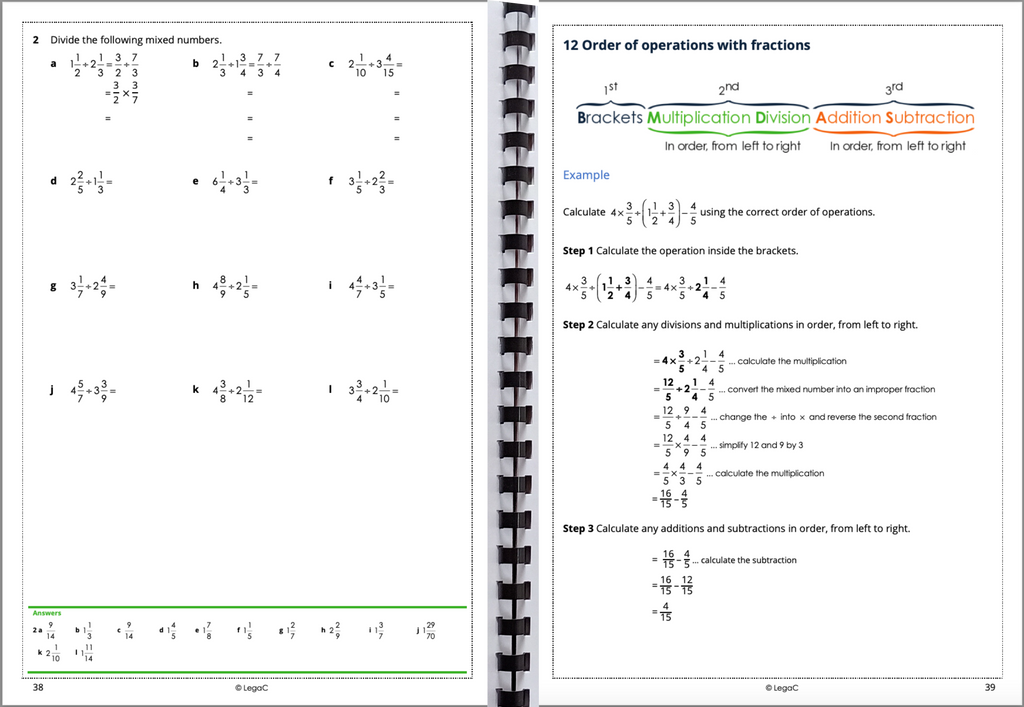Double click for enlarge# Fractions Workbook

Availability: In stock
Quantity:
\$25.00
All you need to know about fractions is in this 50-page book.
* Fraction notation and graphical representations
* Types of fractions and conversions between mixed numbers and
improper fractions
* Equivalent fractions
* Various methods of comparing fractions
* Addition, subtraction, multiplication and division of fractions
* Order of operations with fractions
There are 11 worksheets, a Review set and Problem solving questions.

(0) Items
Items 0
Subtotal \$0.00
To Top